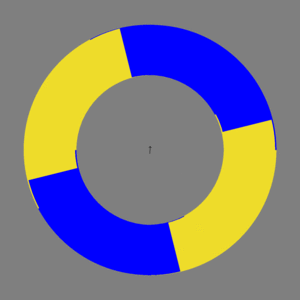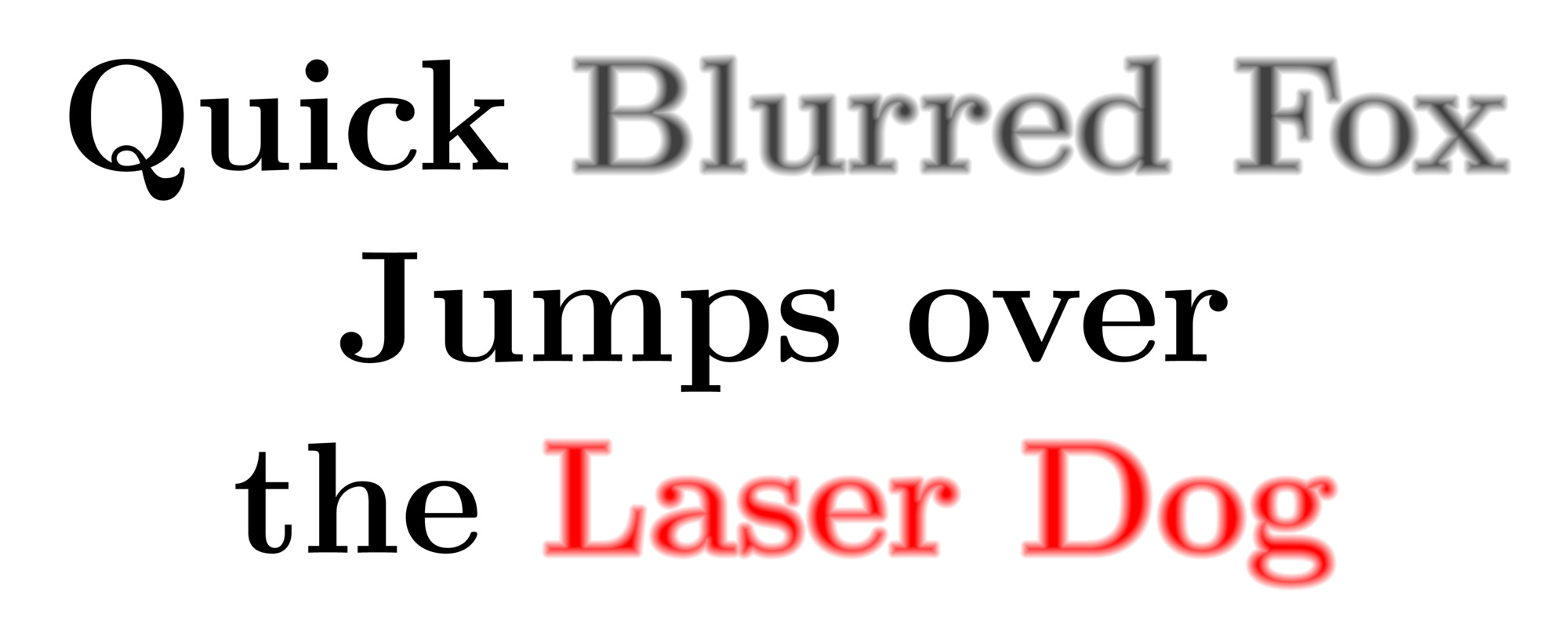# TikZ TeX TalK

## Cycloid% cycloid.tex
\documentclass[tikz]{standalone}
\begin{document}
\def\wheel#1;{
\begin{scope}#1
\clip\pgfextra{\pgfseteorule}circle(5)circle(3);
\fill[yellow!90!black](-9,-9)rectangle(9,9);
\fill[rotate=\f*14,blue](-9,-9)rectangle(0,0)rectangle(9,9);
\end{scope}
}
\foreach\f in{1,...,90}{ % frame
\tikz{
\fill[gray](-6,-6)rectangle(6,6);

\pgfmathsetmacro\dx{0.05*cos(-\f*4)}
\pgfmathsetmacro\dy{0.05*sin(-\f*4)}
\wheel[shift={(\dx,\dy)},rotate=-14]; % help wheel
\wheel[shift={(-\dx,-\dy)},rotate=14]; % help wheel
\wheel[]; % main wheel

\draw[->,scale=3](\dy,-\dx)--(-\dy,\dx);
}
}
\end{document}


Convert pdf to gif with terminal command (with ImageMagick installed)

convert -delay 2 cycloid.pdf cycloid.gif


or with this command that does anti-aliasing

convert -delay 2 -density 300 -resize 300x300 cycloid.pdf cycloid.gif


## Diffuse% diffuse.tex
\documentclass[tikz]{standalone}
\begin{document}
\def\textdiffuse#1{\relax%
\def\diffcoeff{50}%
\pdfliteral{q 1 J 1 j 1 Tr}%
\foreach\mixture in{5,10,...,45}{\relax%
\color{darkgray!\mixture!white}%
\pgfsetlinewidth{(105-2*\mixture)/\diffcoeff}%
\rlap{#1}%
}%
\pdfliteral{q 4 Tr}%
\color{darkgray}%
\rlap{#1}%
\pdfliteral{1 Tr}%
\foreach\mixture in{95,90,...,55}{\relax%
\color{darkgray!\mixture!white}%
\pgfsetlinewidth{(2*\mixture-95)/\diffcoeff}%
\rlap{#1}%
}%
\pdfliteral{Q}%
\pgfsetlinewidth{5/\diffcoeff}%
\color{darkgray!50!white}%
\rlap{#1}%
\pdfliteral{Q}%
\phantom{#1}%
}
\Huge\bfseries
\tikz\node[align=center,scale=10,fill=white]{
Quick \textdiffuse{Blurred Fox}	\\
Jumps over	\\
\colorlet{darkgray}{red}
the \textdiffuse{Laser Dog}
};
\end{document}


Convert pdf to jpg (or png in exchange for file size) with terminal command (with ImageMagick installed)

convert -density 144 -resize 2000 diffuse.pdf diffuse.jpg

Scroll to top